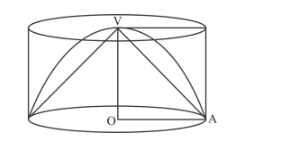# A cone, a hemisphere and a cylinder stand`
Question:

A cone, a hemisphere and a cylinder stand on equal bases and have the same height. What is the ratio of their volumes?

Solution:

Let r be the radius of the base.

And h is the height.

Here, h = r.Now,

The ratio of their volumes will be

Volume of cone: volume of hemisphere: volume of a cylinder

$\frac{1}{3} \pi r^{2} h: \frac{2}{3} \pi r^{3}: \pi r^{2} h$

$V_{1}: V_{2}: V_{3}=\frac{1}{3} \pi r^{3}: \frac{2}{3} \pi r^{3}: \pi r^{3}$

Hence, $V_{1}: V_{2}: V_{3}=1: 2: 3$